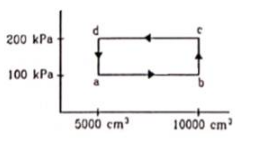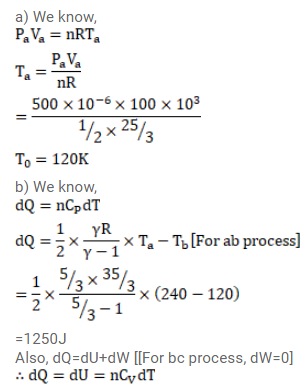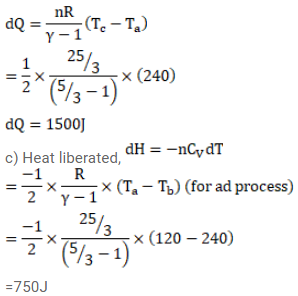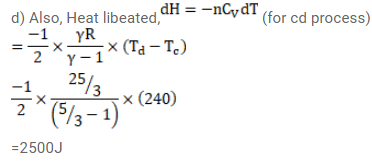# Half mole of an ideal gas (???? = 5/3) is taken throughQuestion:

Half mole of an ideal gas $(\gamma=5 / 3)$ is taken through the cycle abcda as shown in fig. Take $\mathrm{R}=\frac{25}{3} \mathrm{~J} / \mathrm{mol}-\mathrm{K}$ (a) Find the temperature of the gas in the states a,b,c and $d$. (b) Find the amount of heat supplied in the processes ab and bc (c) Find the amount of heat liberated in the processes cd and da.Solution: